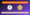# UGC NET Mathematical Reasoning and Aptitude Notes 2020 | Paper 1 Reasoning NotesUGC NET Mathematical Reasoning and Aptitude Notes 2020: Are your preparing for UGC NET Paper 1? Do you have the latest Mathematical Reasoning and Aptitude Notes?

Don’t worry! Here we have provided the latest UGC NET Mathematical Reasoning and Aptitude Notes.

You can check easily check the Mathematical Reasoning and Aptitude Notes and also able to download the PDF.

## UGC NET Mathematical Reasoning and Aptitude Notes 2020

UGC NET Exam, the exam which tests the eligibility of Indian Nationals for ‘Assistant Professor’ or for ‘Junior Research Fellowship and Assistant Professor’ both in Indian Universities and Colleges.

Before starting a complete guide on UGC NET Paper 1 Syllabus 2020 for Environmental Science, you should have an overview of the exam.

UGC NET 2020 June Exam Overview

Here you can check the UGC NET exam overview:

 Exam Name University Grants Commission National Eligibility Test (UGC NET) Conducting Body National Test Agency (NTA) Exam Mode Computer Based Test (CBT) Exam Duration 3 hours Application Mode Online Official Website ugcnet.ntanet.nic.in

Here you can check the complete notes on Mathematical Reasoning and Aptitude In India.

### Number series

Questions on number series are prevalent in most of the exams. Almost 1-2 Questions come in the exam from this topic.

• These questions are based on numerical sequences that follow a logical rule/ pattern based on elementary arithmetic concepts.
• A particular series is given from which the pattern must be analyzed. You are then asked to predict the next number in the sequence following the same rule
• There are some numbers are wrongly put into the series of number and you need to identify or correct them

### UGC NET Reasoning Notes

• Try to analyze the given pattern such as prime numbers, perfect squares, cubes, series of known numbers like Mathematics progression (AP/GP), etc.
• Next..if you are unable to find the familiar number:-
• Calculate difference B/W the given series elements
• Then observe the pattern indifference to see are they following any regular pattern or known numbers like said above.
• If the numbers are growing slowly its must be addition or subtraction series
• If the numbers are growing rapidly it might be a Square series or cube or multiplicative series.
• If the difference or sum doesn’t have any particular pattern it must be an alternate number series.
• Now, You need to t signifies that the series follows a complex
pattern. Check for cases like multiplying the number and adding/subtracting a constant number from it to reach the pattern.

Heads up! There is no defined way to solve numbers series.

The Ultimate trick is to identify the numbers in the series and proceed accordingly.

### How to Identify Common Patterns in Number Series

The common patterns asked in number series topic are-

Arithmetic Series – This could be of two types. in which the next term is obtained by adding/subtracting a constant number to its previous term. “common difference” is the key here

Example: 4, 9, 14, 19 [In the given series pattern is continued by adding 5 to the last number each time]

Furthermore, this could be mixed with Arithmetic Sequences with the differences of consecutive numbers themselves form an arithmetic series.

Example: 1, 3, 6, …..[ in the given series 3 – 1 = 2, 6 – 3 = 3, ..
Now, we get an arithmetic sequence 2, 3,]

Geometric Sequence is multiplying by the same value each time.

Example – 1, 3, 9, 27, … [ The pattern is continued by multiplying by 3 each time…]

Prime numbers-

• Squares/ cubes-
• The pattern in differences-

The pattern in alternate numbers-

As the name of the series specifies, this type of series may consist of two series combined into a single series. The alternating terms of this series may form an independent series in itself.

### Tips and Tricks for Number series for NTA UGC NET EXAM

• Number series questions are mostly numbered in the series are connected to each other. When you identify the connection, solving the question becomes easier for you.
• Identify the pattern asked in the question. Once you identify the pattern, solving the questions would be much easier.
• Once you have identified the pattern, apply it to the number before/ after the missing number in the series to get the answer.

### Other Important UGC NET Paper 1 Notes

You should have the following study materials to boost your exam preparation for the NTA UGC NET exam.

Click on the link to access other important notes related to the UGC NET Paper 1 exam.

We have covered the detailed guide on UGC NET Mathematical Reasoning and Aptitude Notes 2020. Feel free to ask us any questions in the comment section below.